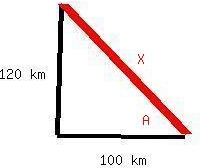# A ship sets out to sail to a point 120 km due north. An unexpected storm blows the ship to a point 100 km due east of its starting point. (a) How far and (b) in what direction must it now sail to reach its original destination?bobbie71G 2020-12-16 Answered
A ship sets out to sail to a point 120 km due north. An unexpected storm blows the ship to a point 100 km due east of its starting point. (a) How far and (b) in what direction must it now sail to reach its original destination?
You can still ask an expert for help

• Questions are typically answered in as fast as 30 minutes

Solve your problem for the price of one coffee

• Math expert for every subject
• Pay only if we can solve itbahaistaga) Use the Pythagorean theorem: ${C}^{2}={A}^{2}+{B}^{2}$
$\text{distance}=\sqrt{{100}^{2}+{120}^{2}}$
b) as for direction, the ship must sail northwest

###### Not exactly what you’re looking for?Jeffrey Jordon

Step 1

As the storm will be blowing the ship 100km to the east, the ship has to move 100km west to overcome the effect of ship

Also to reach the destination, the ship has to move 120km to its north. Thus in total the sailor has to more 120km north and 100km west

Thus

$D=-100\stackrel{^}{i}+120\stackrel{^}{j}$

considering positive $\stackrel{^}{i}$ towards east and positive $\stackrel{^}{j}$ towards north

a)As we know that the directions north and east are perpendicular to each other, the total distance the ship has to make can be calculated as shown below

${D}^{2}={120}^{2}+{100}^{2}$

${D}^{2}=14400+10000$

${D}^{2}=24400$

D=156.2km

b)As explained above, the ship has to move 100km to the west and 120km to the north,

$D=-100\stackrel{^}{i}+120\stackrel{^}{j}$

thus the direction in which the ship has to move will be

$\theta =\mathrm{arctan}\frac{120}{-100}$

$\theta =\mathrm{arctan}\frac{120}{-100}$ (value has to be in 2nd quadrant as $\stackrel{^}{i}$  has negative value and  $\stackrel{^}{j}$  has positive value)

$\theta =\mathrm{arctan}-1.2$

$\theta ={129.8}^{\circ }$